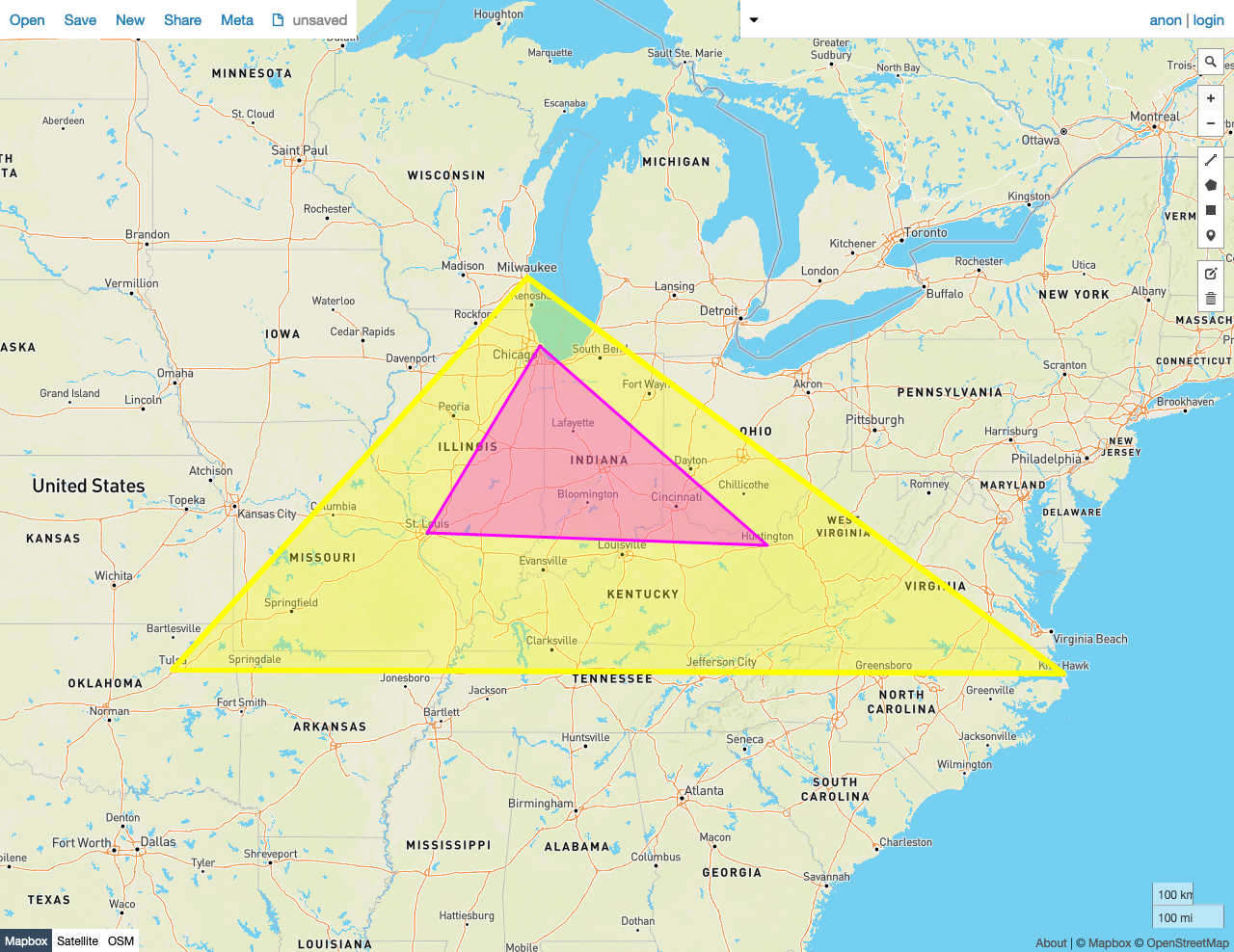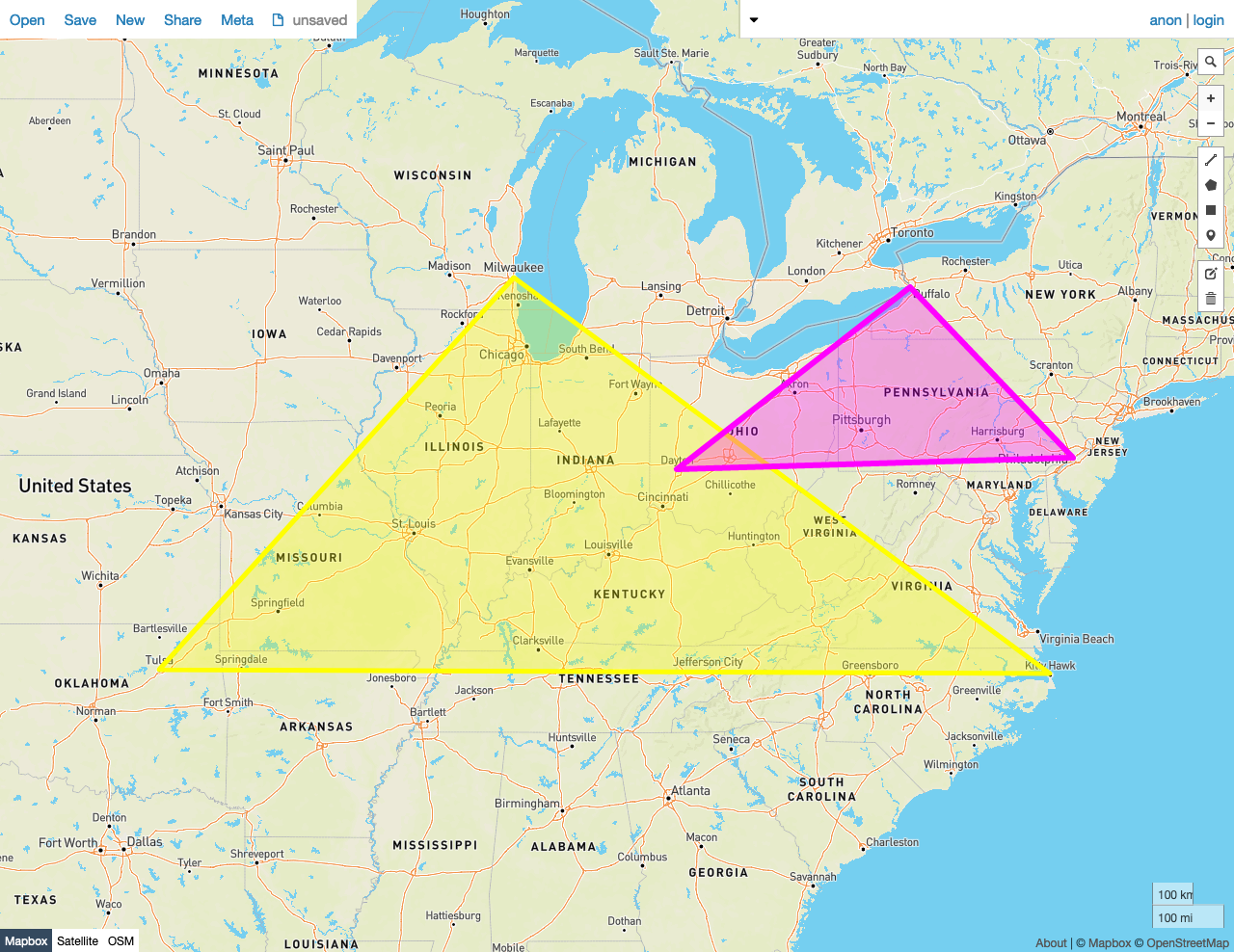Given two shapes A and B, predicate function ST_Covers(A, B) returns true if no point in B lies outside of shape A. Otherwise, it returns false.

In other words, shape A must completely cover every point in B.

This behavior is similar to ST_Contains, except that the criteria for ST_Contains are more exacting, and therefore some pairs of shapes will be accepted by this function that would be rejected by ST_Contains.

ST_Covers works on the following data types:

Note:

ST_Covers will attempt to use any available spatial index to speed up its operation. Use the prefixed variant _ST_Covers if you do not want any spatial indexes to be used.

Note:

This function is the inverse of ST_CoveredBy.

Examples

Note:

The screenshots in these examples were generated using geojson.io, but they are designed to showcase the shapes, not the map. Representing GEOMETRY data in GeoJSON can lead to unexpected results if using geometries with SRIDs other than 4326 (as shown below).

True

In this example, ST_Covers returns true because:

• No Point in the smaller Polygon B lies outside of the larger Polygon A.
SELECT ST_Covers(st_geomfromtext('SRID=4326;POLYGON((-87.906471 43.038902, -95.992775 36.153980, -75.704722 36.076944, -87.906471 43.038902))'), st_geomfromtext('SRID=4326;POLYGON((-87.623177 41.881832, -90.199402 38.627003, -82.446732 38.413651, -87.623177 41.881832))'));
st_covers
---------------
true

(1 row)False

In this example, ST_Covers returns false because:

• Many Points in the smaller Polygon B lie outside of the larger Polygon A.
SELECT ST_Covers(st_geomfromtext('SRID=4326;POLYGON((-87.906471 43.038902, -95.992775 36.153980, -75.704722 36.076944, -87.906471 43.038902))'), st_geomfromtext('SRID=4326;POLYGON((-84.191605 39.758949, -75.165222 39.952583, -78.878738 42.880230, -84.191605 39.758949))'));
st_covers
---------------
false
(1 row)